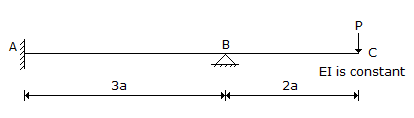# Civil Engineering - UPSC Civil Service Exam Questions

21.

If the stream function ψ = 3x2 - y3, what is the magnitude of velocity at point (2, 2) ?

 A. 9 B. 13 C. 15 D. 17

Explanation:

No answer description available for this question. Let us discuss.

22.

In each of the following examples certain quantity of work 'W' is done.
1. The point of application of a force of (50i + 60j + 80k) in kN units moves through a distance given by (6i + 5j + 2.5k) in metres.
2. A pump raises 2m3 of water through a height of 20 m (Take g = 10 m/s2).
3. A shaft delivering a torque = 200 kNm makes 0.75 revolution.
4. A couple (30i - 20j + 100k) in kNm units acting on a body rotates it through an angular displacement of (2i + 3j + 6k) radians.
The correct sequence of these quantities of work 'W' arranged in order of their increasing magnitude is

 A. 1, 4, 2, 3 B. 2, 4, 1, 3 C. 3, 2, 1, 4 D. 2, 3, 4, 1

Explanation:

No answer description available for this question. Let us discuss.

23.

Which one of the following characteristics of ballast makes it unsuitable for use ?

 A. High resilience B. High stability C. High water absorption D. High modulus

Explanation:

No answer description available for this question. Let us discuss.

24.

In the propped cantilever shown in the figure below, what are the values of the bending moment and shear force at the support A ?A. 2 Pa, P/3 B. Pa, P C. Pa, P/3 D. 2 Pa, 4 P/3

Explanation:

No answer description available for this question. Let us discuss.

25.

Which one of the following methods of O-D traffic surveys is conducted for comprehensive analysis of traffic and transportation data ?

 A. Home interview B. Roadside interview C. Registration number method D. Postcard method